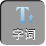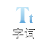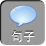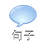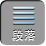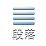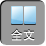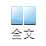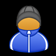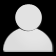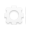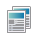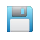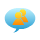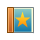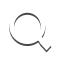-AA+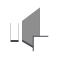1.设集合 A={ x| 1x4}，集合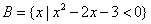, 则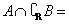（ ）

A.( 1,4) B.( 3,4) C.( 1,3) D.( 1,2)∪（ 3,4

【答案】 B

【解析】=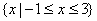{ x| 1x4}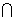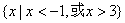=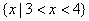故选 B.

2.已知集合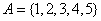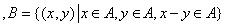；,则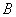中所含元素的个数为（ ）

A. 3 B. 6 C. 8 D. 10

【答案】 D

【解析】要使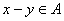,当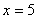时，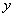可是 1234.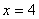时，可是 123.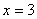时，可是 12.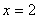时，可是 1，综上共有 10个，选 D.

3.已知全集 U=｛ 0,1,2,3,4,5,6,7,8,9｝，集合 A=｛ 0,1,3,5,8｝，集合 B=｛ 2,4,5,6,8｝，则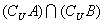为（ ）

A. { 5,8} B. { 7,9} C. { 0,1,3} D. { 2,4,6}

【答案】 B

【解析】因为全集 U=｛ 0,1,2,3,4,5,6,7,8,9｝，集合 A=｛ 0,1,3,5,8｝，集合 B=｛ 2,4,5,6,8｝，所以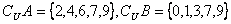，所以为{ 7,9}.故选 B

4.若集合 A=｛ -11｝， B=｛ 02｝，则集合｛ zz= x+ y, xA, yB｝中的元素的个数为（）

A5 B. 4 C. 3 D. 2

【答案】 C

【解析】因为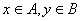，所以当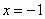时，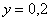，此时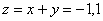时，，此时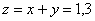，所以集合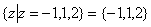共三个元素，选 C.

5.设集合 M={ -1,0,1}， N={ x| x 2x}，则 MN=()

A. { 0} B. { 0, 1} C. { -1,1} D. { -1,0,0}

【答案】 B

【解析】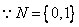M={ -1,0,1}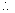MN={ 0,1}.

【点评】本题考查了集合的基本运算，较简单，易得分先求出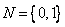,再利用交集定义得出 MN.

6.设集合 A={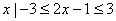}，集合 B为函数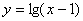的定义域，则 A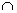B=（）

A. 12B. [ 12] C. [ 12D. 12]

【答案】 D

【解析】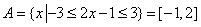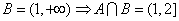.

7.已知集合 A={ x| x 2x2< 0}， B={ x|－ 1< x< 1}，则（）

A. A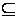B B. BA C. A= B D. BA

【答案】 B

【解析】集合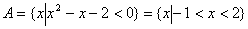，又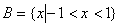，所以 BA的真子集，选 B.

1.已知集合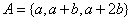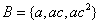．若 A= B，则 c的值是______．

【答案】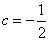.

【解析】要解决 c的求值问题，关键是要有方程的数学思想，此题应根据相等的两个集合元素完全相同及集合中元素的确定性、互异性，无序性建立关系式．

1）若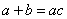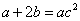，消去 b得：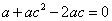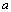= 0时，集合 B中的三元素均为零，和元素的互异性相矛盾，故0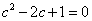，即 c= 1，但 c= 1时， B中的三元素又相同，此时无解．

2）若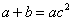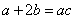，消去 b得：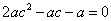a0，∴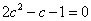，即( c1)( 2 c1)= 0，又 c1，故 c=－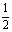2.已知集合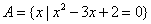,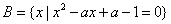，且 AB= A，则 a的值为______．

【答案】 a的值为 23.

【解析】由 AB= A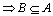而推出 B有四种可能，进而求出 a的值．

AB= A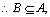A={ 12}，∴ B=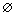B={ 1}或 B={ 2}或 B={ 12}．

B=，则令△< 0 aB={ 1}，则令△= 0 a= 2，此时 1是方程的根；

B={ 2}，则令△= 0 a= 2，此时 2不是方程的根，∴ aB={ 12}则令△> 0 aR a2，把 x= 1代入方程得 aR，把 x= 2代入方程得 a= 3